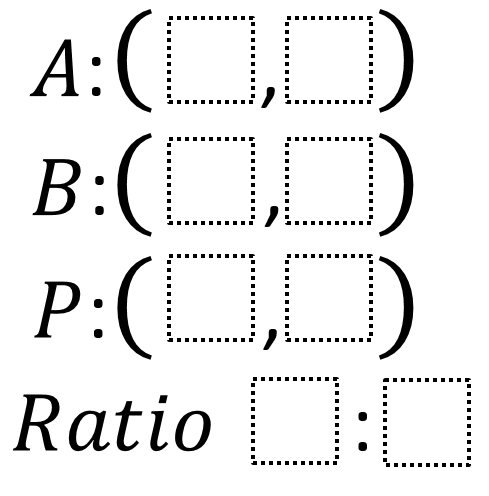# Partitioning a Line Segment

Directions: Using the digits 1 to 8 exactly one time each, fill in the boxes to create a line segment AB, where between point A and point B, there exists a point P so that it partitions line segment AB into a ratio.### Hint

How do you know if a point is on a line? Are there certain lines or points that are “easier” to deal with?

A (2,4), B (6,8), P (5,7) Ratio is 3:1

Source: Jon Henderson

## Exponential Powers

Directions: Using the digits 0 to 9 at most one time each, place a digit …

### One comment

1.A= (2,4) B=(6,8), P(5,7)
The ratio is 3:1